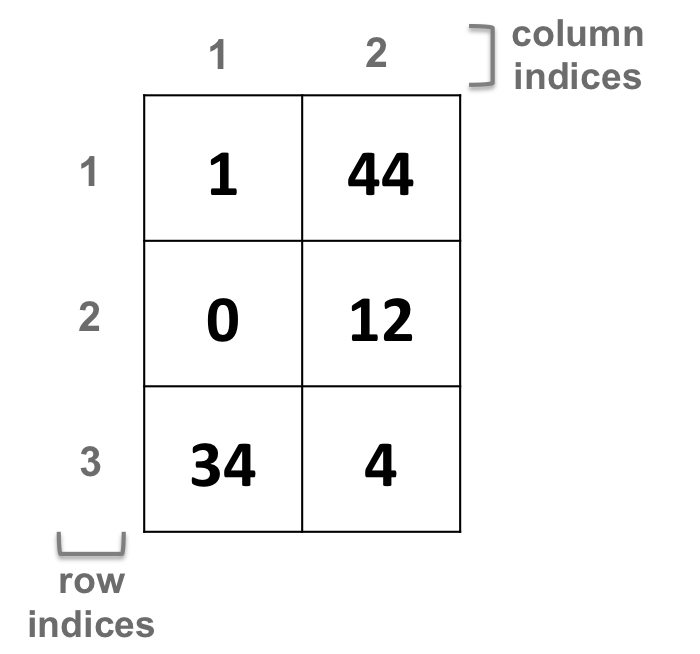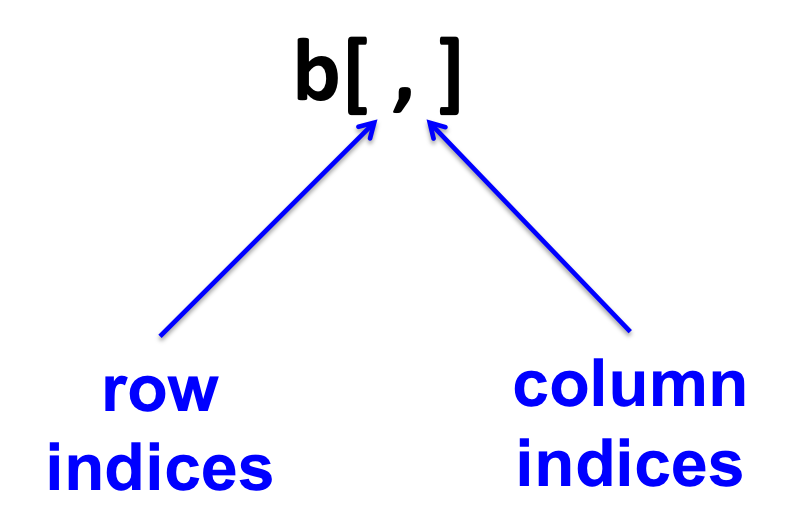## 9.5 Matrices

• A matrix is a 2 dimensional vector.

• All columns in a matrix must have:
• the same type (numeric, character or logical)
• the same length

### 9.5.1 Creating a matrix

• From vectors with the rbind function:
``````x <- c(1, 44)
y <- c(0, 12)
z <- c(34, 4)
# rbind: bind rows
b <- rbind(x, y, z)``````
• From vectors with the cbind function:
``````i <- c(1, 0, 34)
j <- c(44, 12, 4)
# cbind: bind columns
b <- cbind(i, j)``````
• From scratch with the matrix function:
``````# nrow: number of rows
# ncol: number of columns
b <- matrix(c(1, 0, 34, 44, 12, 4),
nrow=3,
ncol=2)``````

### 9.5.2 Two-dimensional object

Vectors have one index per element (1-dimension).
Matrices have two indices (2-dimensions) per element, corresponding to the row and the column:• Fetching elements of a matrix:

The “coordinates” of an element in a 2-dimensional object will be first the row (on the left of the comma), then the column (on the right of the comma):### 9.5.3 Matrix manipulation

• Add 1 to all elements of a matrix
``b <- b + 1``
• Multiply by 3 all elements of a matrix
``b <- b * 3``
• Subtract 2 to each element of the first row of a matrix
``b[1, ] <- b[1, ] - 2``
• Replace elements that comply a condition:
``````# Replace all elements that are greater than 3 with NA
b[ b>3 ] <- NA``````